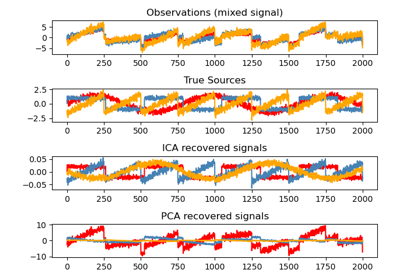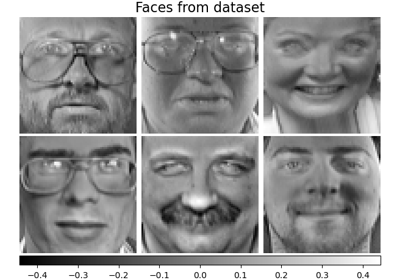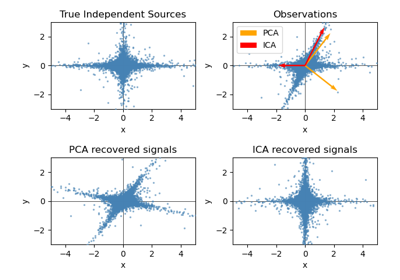# sklearn.decomposition.FastICA¶

class sklearn.decomposition.FastICA(n_components=None, *, algorithm='parallel', whiten='unit-variance', fun='logcosh', fun_args=None, max_iter=200, tol=0.0001, w_init=None, whiten_solver='svd', random_state=None)[source]

FastICA: a fast algorithm for Independent Component Analysis.

The implementation is based on .

Read more in the User Guide.

Parameters:
n_componentsint, default=None

Number of components to use. If None is passed, all are used.

algorithm{‘parallel’, ‘deflation’}, default=’parallel’

Specify which algorithm to use for FastICA.

whitenstr or bool, default=’unit-variance’

Specify the whitening strategy to use.

• If ‘arbitrary-variance’, a whitening with variance arbitrary is used.

• If ‘unit-variance’, the whitening matrix is rescaled to ensure that each recovered source has unit variance.

• If False, the data is already considered to be whitened, and no whitening is performed.

Changed in version 1.3: The default value of whiten changed to ‘unit-variance’ in 1.3.

fun{‘logcosh’, ‘exp’, ‘cube’} or callable, default=’logcosh’

The functional form of the G function used in the approximation to neg-entropy. Could be either ‘logcosh’, ‘exp’, or ‘cube’. You can also provide your own function. It should return a tuple containing the value of the function, and of its derivative, in the point. The derivative should be averaged along its last dimension. Example:

def my_g(x):
return x ** 3, (3 * x ** 2).mean(axis=-1)

fun_argsdict, default=None

Arguments to send to the functional form. If empty or None and if fun=’logcosh’, fun_args will take value {‘alpha’ : 1.0}.

max_iterint, default=200

Maximum number of iterations during fit.

tolfloat, default=1e-4

A positive scalar giving the tolerance at which the un-mixing matrix is considered to have converged.

w_initarray-like of shape (n_components, n_components), default=None

Initial un-mixing array. If w_init=None, then an array of values drawn from a normal distribution is used.

whiten_solver{“eigh”, “svd”}, default=”svd”

The solver to use for whitening.

• “svd” is more stable numerically if the problem is degenerate, and often faster when n_samples <= n_features.

• “eigh” is generally more memory efficient when n_samples >= n_features, and can be faster when n_samples >= 50 * n_features.

New in version 1.2.

random_stateint, RandomState instance or None, default=None

Used to initialize w_init when not specified, with a normal distribution. Pass an int, for reproducible results across multiple function calls. See Glossary.

Attributes:
components_ndarray of shape (n_components, n_features)

The linear operator to apply to the data to get the independent sources. This is equal to the unmixing matrix when whiten is False, and equal to np.dot(unmixing_matrix, self.whitening_) when whiten is True.

mixing_ndarray of shape (n_features, n_components)

The pseudo-inverse of components_. It is the linear operator that maps independent sources to the data.

mean_ndarray of shape(n_features,)

The mean over features. Only set if self.whiten is True.

n_features_in_int

Number of features seen during fit.

New in version 0.24.

feature_names_in_ndarray of shape (n_features_in_,)

Names of features seen during fit. Defined only when X has feature names that are all strings.

New in version 1.0.

n_iter_int

If the algorithm is “deflation”, n_iter is the maximum number of iterations run across all components. Else they are just the number of iterations taken to converge.

whitening_ndarray of shape (n_components, n_features)

Only set if whiten is ‘True’. This is the pre-whitening matrix that projects data onto the first n_components principal components.

PCA

Principal component analysis (PCA).

IncrementalPCA

Incremental principal components analysis (IPCA).

KernelPCA

Kernel Principal component analysis (KPCA).

MiniBatchSparsePCA

Mini-batch Sparse Principal Components Analysis.

SparsePCA

Sparse Principal Components Analysis (SparsePCA).

References



A. Hyvarinen and E. Oja, Independent Component Analysis: Algorithms and Applications, Neural Networks, 13(4-5), 2000, pp. 411-430.

Examples

>>> from sklearn.datasets import load_digits
>>> from sklearn.decomposition import FastICA
>>> transformer = FastICA(n_components=7,
...         random_state=0,
...         whiten='unit-variance')
>>> X_transformed = transformer.fit_transform(X)
>>> X_transformed.shape
(1797, 7)


Methods

 fit(X[, y]) Fit the model to X. fit_transform(X[, y]) Fit the model and recover the sources from X. get_feature_names_out([input_features]) Get output feature names for transformation. Get metadata routing of this object. get_params([deep]) Get parameters for this estimator. inverse_transform(X[, copy]) Transform the sources back to the mixed data (apply mixing matrix). set_inverse_transform_request(*[, copy]) Request metadata passed to the inverse_transform method. set_output(*[, transform]) Set output container. set_params(**params) Set the parameters of this estimator. set_transform_request(*[, copy]) Request metadata passed to the transform method. transform(X[, copy]) Recover the sources from X (apply the unmixing matrix).
fit(X, y=None)[source]

Fit the model to X.

Parameters:
Xarray-like of shape (n_samples, n_features)

Training data, where n_samples is the number of samples and n_features is the number of features.

yIgnored

Not used, present for API consistency by convention.

Returns:
selfobject

Returns the instance itself.

fit_transform(X, y=None)[source]

Fit the model and recover the sources from X.

Parameters:
Xarray-like of shape (n_samples, n_features)

Training data, where n_samples is the number of samples and n_features is the number of features.

yIgnored

Not used, present for API consistency by convention.

Returns:
X_newndarray of shape (n_samples, n_components)

Estimated sources obtained by transforming the data with the estimated unmixing matrix.

get_feature_names_out(input_features=None)[source]

Get output feature names for transformation.

The feature names out will prefixed by the lowercased class name. For example, if the transformer outputs 3 features, then the feature names out are: ["class_name0", "class_name1", "class_name2"].

Parameters:
input_featuresarray-like of str or None, default=None

Only used to validate feature names with the names seen in fit.

Returns:
feature_names_outndarray of str objects

Transformed feature names.

Get metadata routing of this object.

Please check User Guide on how the routing mechanism works.

Returns:

A MetadataRequest encapsulating routing information.

get_params(deep=True)[source]

Get parameters for this estimator.

Parameters:
deepbool, default=True

If True, will return the parameters for this estimator and contained subobjects that are estimators.

Returns:
paramsdict

Parameter names mapped to their values.

inverse_transform(X, copy=True)[source]

Transform the sources back to the mixed data (apply mixing matrix).

Parameters:
Xarray-like of shape (n_samples, n_components)

Sources, where n_samples is the number of samples and n_components is the number of components.

copybool, default=True

If False, data passed to fit are overwritten. Defaults to True.

Returns:
X_newndarray of shape (n_samples, n_features)

Reconstructed data obtained with the mixing matrix.

set_inverse_transform_request(*, copy: Union[bool, None, str] = '$UNCHANGED$') [source]

Request metadata passed to the inverse_transform method.

Note that this method is only relevant if enable_metadata_routing=True (see sklearn.set_config). Please see User Guide on how the routing mechanism works.

The options for each parameter are:

• True: metadata is requested, and passed to inverse_transform if provided. The request is ignored if metadata is not provided.

• False: metadata is not requested and the meta-estimator will not pass it to inverse_transform.

• None: metadata is not requested, and the meta-estimator will raise an error if the user provides it.

• str: metadata should be passed to the meta-estimator with this given alias instead of the original name.

The default (sklearn.utils.metadata_routing.UNCHANGED) retains the existing request. This allows you to change the request for some parameters and not others.

New in version 1.3.

Note

This method is only relevant if this estimator is used as a sub-estimator of a meta-estimator, e.g. used inside a Pipeline. Otherwise it has no effect.

Parameters:
copystr, True, False, or None, default=sklearn.utils.metadata_routing.UNCHANGED

Metadata routing for copy parameter in inverse_transform.

Returns:
selfobject

The updated object.

set_output(*, transform=None)[source]

Set output container.

See Introducing the set_output API for an example on how to use the API.

Parameters:
transform{“default”, “pandas”}, default=None

Configure output of transform and fit_transform.

• "default": Default output format of a transformer

• "pandas": DataFrame output

• None: Transform configuration is unchanged

Returns:
selfestimator instance

Estimator instance.

set_params(**params)[source]

Set the parameters of this estimator.

The method works on simple estimators as well as on nested objects (such as Pipeline). The latter have parameters of the form <component>__<parameter> so that it’s possible to update each component of a nested object.

Parameters:
**paramsdict

Estimator parameters.

Returns:
selfestimator instance

Estimator instance.

set_transform_request(*, copy: Union[bool, None, str] = '$UNCHANGED$') [source]

Request metadata passed to the transform method.

Note that this method is only relevant if enable_metadata_routing=True (see sklearn.set_config). Please see User Guide on how the routing mechanism works.

The options for each parameter are:

• True: metadata is requested, and passed to transform if provided. The request is ignored if metadata is not provided.

• False: metadata is not requested and the meta-estimator will not pass it to transform.

• None: metadata is not requested, and the meta-estimator will raise an error if the user provides it.

• str: metadata should be passed to the meta-estimator with this given alias instead of the original name.

The default (sklearn.utils.metadata_routing.UNCHANGED) retains the existing request. This allows you to change the request for some parameters and not others.

New in version 1.3.

Note

This method is only relevant if this estimator is used as a sub-estimator of a meta-estimator, e.g. used inside a Pipeline. Otherwise it has no effect.

Parameters:
copystr, True, False, or None, default=sklearn.utils.metadata_routing.UNCHANGED

Metadata routing for copy parameter in transform.

Returns:
selfobject

The updated object.

transform(X, copy=True)[source]

Recover the sources from X (apply the unmixing matrix).

Parameters:
Xarray-like of shape (n_samples, n_features)

Data to transform, where n_samples is the number of samples and n_features is the number of features.

copybool, default=True

If False, data passed to fit can be overwritten. Defaults to True.

Returns:
X_newndarray of shape (n_samples, n_components)

Estimated sources obtained by transforming the data with the estimated unmixing matrix.

## Examples using sklearn.decomposition.FastICA¶Blind source separation using FastICA

Blind source separation using FastICAFaces dataset decompositions

Faces dataset decompositionsFastICA on 2D point clouds

FastICA on 2D point clouds